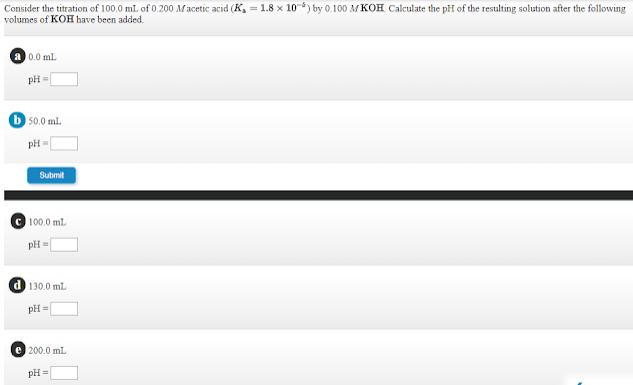# Consider the titration of 100.0 mL of 0.200 M acetic acid (Ka = 1.8 x 10^-5) by 0.100 M KOH. Calculate the pH of the resulting solution after the following volumes of KOH have been added. a. 0.0 mL pH = ___ b. 50.0 mL pH = ___ c. 100.0 mL pH = ___ d. 130.0 mL = ___ e. 200.0 mL pH = ___